Question

an experimenter flips a coin 100 times and gets 54 heads. Test the claim that the coin is fair against the two-sided alternative.

Given that coin is flips 100 times and gets 54 heads.

x = 54 and n = 100

we have to test,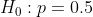verses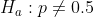Under Ho test statistic is,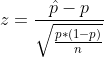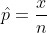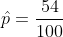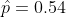So,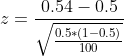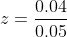So test statistic is Z = 0.8

p-value is given by,

P-value = 2*P(Z >0.8) = 2*0.21 =0.42

So p-vlaue = 0.42 > 0.05 so do not reject null hypothesis

So We conclude that The coin is fair .

#### Earn Coins

Coins can be redeemed for fabulous gifts.

Similar Homework Help Questions
• ### An experimenter flips a coin 100 times and gets 44 heads. Test the claim that the...

An experimenter flips a coin 100 times and gets 44 heads. Test the claim that the coin is fair against the two-sided claim that it is not fair at the level α=.01

• ### Question 9 An experimenter flips a coin 100 times and gets 58 heads. Test the claim...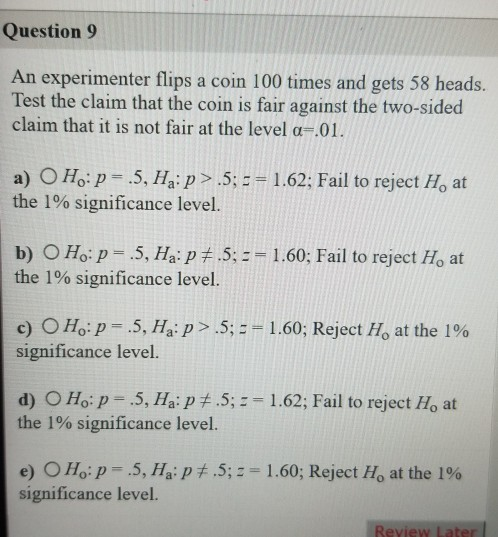Question 9 An experimenter flips a coin 100 times and gets 58 heads. Test the claim that the coin is fair against the two-sided claim that it is not fair at the level a=.01. a) O H.:p= .5, Ha:p> .5; = = 1.62; Fail to reject H, at the 1% significance level. b) O Ho: p= .5, Ha:p #.5; == 1.60; Fail to reject H, at the 1% significance level. c) O H.:p= .5, H.:P > .5; == 1.60; Reject...

• ### Tegan is trying to decide if a coin is fair. She flips it 100 times...

Tegan is trying to decide if a coin is fair. She flips it 100 times and gets 63 heads. Please explain why it might make sense to view 63 heads as enough evidence to conclude the coin is unfair.

• ### Leela is trying to decide if a coin is fair. She flips it 100 times and...

Leela is trying to decide if a coin is fair. She flips it 100 times and gets 63 heads. Please explain why it might NOT make sense to view 63 heads as enough evidence to conclude the coin is unfair.

• ### Polly is testing a coin to see if it is fair. She flips it 100 times...

Polly is testing a coin to see if it is fair. She flips it 100 times and gets 50 heads. What should her conclusion be?

• ### You flip a coin 100 times. Let X= the number of heads in 100 flips. Assume...

You flip a coin 100 times. Let X= the number of heads in 100 flips. Assume we don’t know the probability, p, the coin lands on heads (we don’t know its a fair coin). So, let Y be distributed uniformly on the interval [0,1]. Assume the value of Y = the probability that the coin lands on heads. So, we are given Y is uniformly distributed on [0,1] and X given Y=p is binomially distributed on (100,p). Find E(X) and...

• ### You flip the same coin 90 mores times (100 total flips). If half of the 90...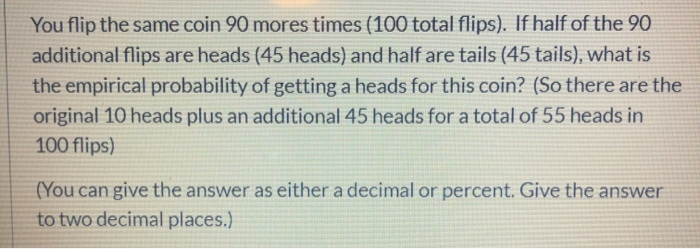You flip the same coin 90 mores times (100 total flips). If half of the 90 additional flips are heads (45 heads) and half are tails (45 tails), what is the empirical probability of getting a heads for this coin? (So there are the original 10 heads plus an additional 45 heads for a total of 55 heads in 100 flips) (You can give the answer as either a decimal or percent. Give the answer to two decimal places.)

• ### Consider successive flips of a fair coin,...

Consider successive flips of a fair coin, making the usual assumptions of independent outcomes on the various flips. (Note: By “fair coin” it is meant that Heads andTails are equally likely.)(a) If the coin is flipped five times, what is the probability that heads occurs all five times?(b) If the coin is flipped just twice, what is the probability that both flips result in heads given that at least one of the flips results in heads?

• ### 3. Consider a coin-die experiment: One flips a fair coin at first. If he gets a...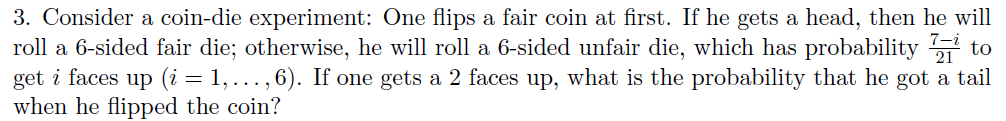3. Consider a coin-die experiment: One flips a fair coin at first. If he gets a head, then he will roll a 6-sided fair die; otherwise, he will roll a 6-sided unfair die, which has probability to get i faces up (i = i, . . . , 6). If one gets a 2 faces up, what is the probability that he got a tail when he flipped the coin? 2.1

• ### 1. A fair coin is flipped four times. Find the probability that exactly two of the...

1. A fair coin is flipped four times. Find the probability that exactly two of the flips will turn up as heads. 2. A fair coin is flipped four times. Find the probability that at least two of the flips will turn up as heads. 3. A six-sided dice is rolled twice. Find the probability that the larger of the two rolls was equal to 3. 4. A six-sided dice is rolled twice. Find the probability that the larger of...